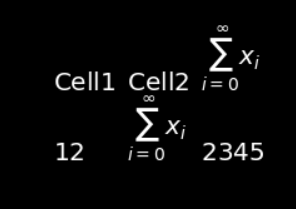# MatplotlibDeprecationWarning when render math equation

I want to render math equation in my application. The minimal code is:

import vtkmodules.all as vtk

text = vtk.vtkTextActor()
text.SetInput('Cell1 | Cell2 | $\\sum_{i=0}^\\infty x_i$\n12 | $\\sum_{i=0}^\\infty x_i$ | 2345')
text.SetDisplayPosition(100, 100)
property = text.GetTextProperty()
property.SetFontSize(20)

render = vtk.vtkRenderer()
renWin = vtk.vtkRenderWindow()
iren = vtk.vtkRenderWindowInteractor()
iren.SetRenderWindow(renWin)
iren.Initialize()
iren.Start()


It can correctly render the math equation as following:However, it also report a warning:

MatplotlibDeprecationWarning:
The MathtextBackendBitmap class was deprecated in Matplotlib 3.4 and will be removed two minor releases later. Use mathtext.math_to_image instead.
iren.Start()


Why this warning is reported?

The environment is:

win 10
python 3.9.12
vtk 9.1.0
matplotlib 3.5.1


It seems that VTK implementation uses a deprecated matplotlib function. The warning tells us where the problem is and how to fix it. Please file a bug report with this warning
https://gitlab.kitware.com/vtk/vtk/-/issues

Dan